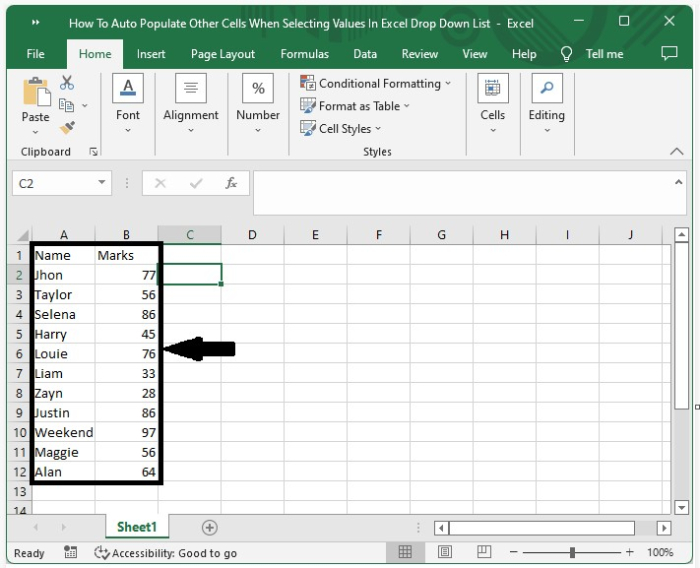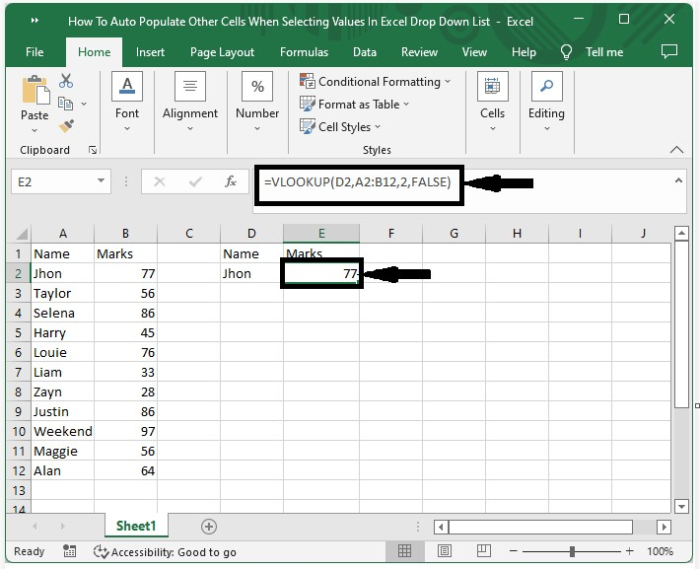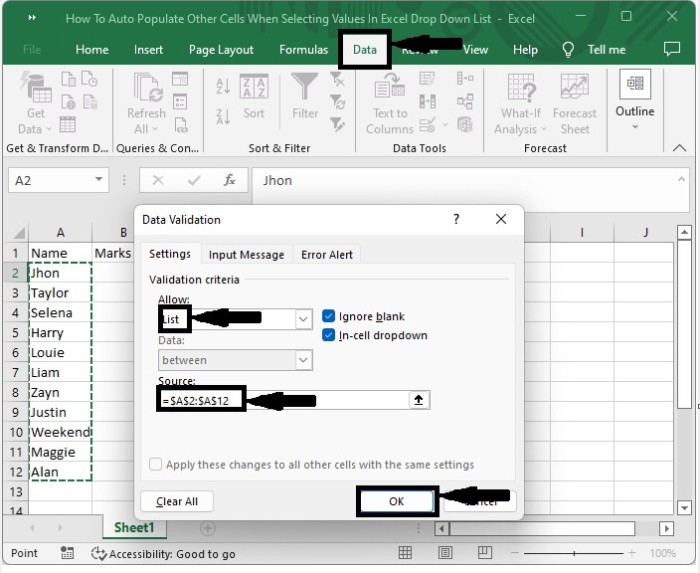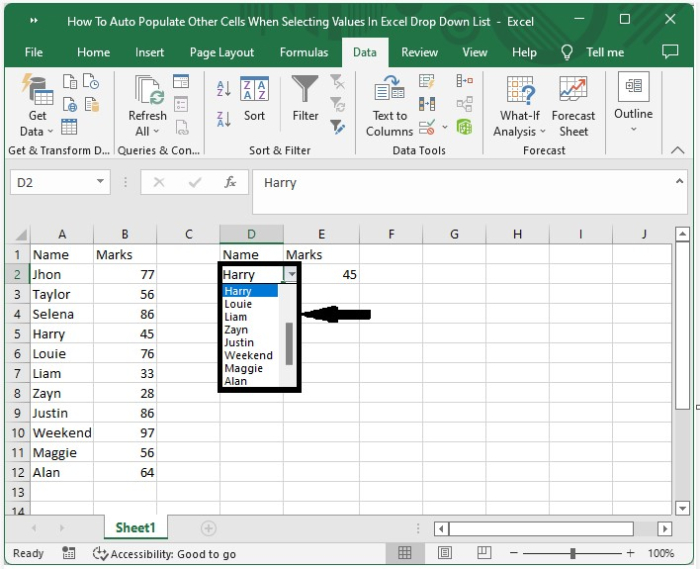# How to Auto-Populate Other Cells When Selecting Values in an Excel Dropdown List?

Have you ever tried to get the values of an object using a drop-down list so that the value represented in the cell is based on the value we select from the drop-down list? This can be done using the process mentioned in this article.

Read this tutorial to learn how you can autopopulate other values when selecting values in an Excel drop-down list. Mainly, this process has two parts: the first part is to use the VLOOKUP function, and the next step is creating a drop-down list.

Here we will first get the list of marks using the VLOOKUP function and then create a data validation list for names. Let us see a simple process to understand how to auto-populate other cells when selecting values from a drop-down list in Excel.

Step 1

Let us consider an Excel sheet where the data in the sheet is similar to the data present in the below image.Now, in cell D2, duplicate the value of cell A2 and enter the formula =VLOOKUP(D2,A2:B12,2,FALSE) in cell E2 and click Enter. Our result will be similar to the data shown in the image below.Step 2

We have got the first result; now we need to create the drop-down list in the cell D2.

To create the drop-down list, click on cell D2 and click on data, then select data validation. A new pop-up window will be opened as shown in the below image.Step 3

Select "Allow to List" and "Source as: cells A2 to A12" in the new pop-up window, then click "OK" to successfully get our drop-down list, from which we will be able to determine the value of cell E2.## Conclusion

In this tutorial, we used a simple example to demonstrate how we can auto-populate other cells by selecting the values from a drop-down list in Excel.

Updated on: 11-Jan-2023

7K+ Views